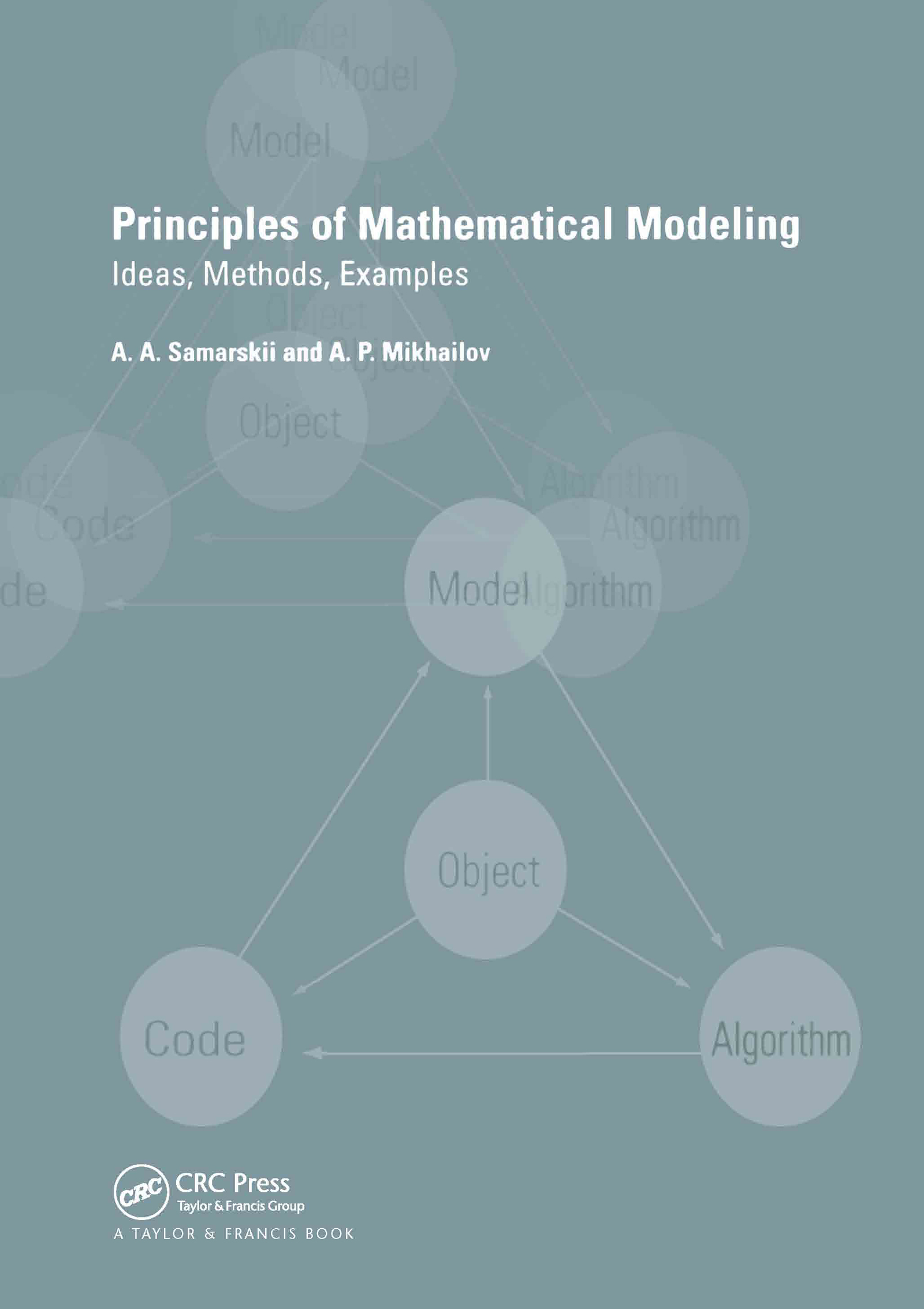Principles of Mathematical Modelling

Ideas, Methods, Examples, 1st Edition

CRC Press

360 pages

Purchasing Options:\$ = USD
Paperback: 9780415272810
pub: 2001-12-20
Currently out of stock
\$135.00
x
eBook (VitalSource) : 9780429165436
pub: 2001-12-20
from \$65.00

FREE Standard Shipping!

Description

Mathematical modeling is becoming increasingly versatile and multi-disciplinary. This text demonstrates the broadness of this field as the authors consider the principles of model construction and use common approaches to build models from a range of subject areas. The book reflects the interests and experiences of the authors, but it explores mathematical modeling across a wide range of applications, from mechanics to social science. A general approach is adopted, where ideas and examples are favored over rigorous mathematical procedures. This insightful book will be of interest to specialists, teachers, and students across a wide range of disciplines..

Elementary Mathematical Models. Examples of Models Following from the Fundamental Laws of Nature. Variational Principles and Mathematical Models. Example of the Hierarchy of Models. The Universality of Mathematical Models. Several Models of Elementary Nonlinear Objects. Derivation of Models from the Fundamental Laws of Nature. Conservation of the Mass of Substance. Conservation of Energy. Conservation of the Number of Particles. Joint Application of Several Fundamental Laws. Models Deduced from Variational Principles, Hierarchies of Models. Equations of Motion, Variational Principles and Conservation Laws in Mechanics. Models of Some Mechanical Systems. The Boltzmann Equation and its Derivative Equations. Models of Some Hardly Formalizable Objects. Universality of Mathematical Models. Some Models of Financial and Economic Processes. Some Rivalry Models. Dynamics of Distribution of Power in Hierarchy. Application of Similarity Methods. The Maximum Principle and Comparison Theorems. An Averaging Method. On Transition to Discrete Models. Mathematical Modeling of Complex Objects. Problems of Technology and Ecology. Fundamental Problems of Natural Science. Computing Experiment With Models of Hardly Formalizable Objects. References. Index.

Professor Alexander A. Samarskii is Director of the Institute of Mathematical Modelling at the Russian Academy of Science and has been the leader of the Russian National Program for Mathematical Modelling in Science and Technology since 1985. He is a world-renowned researcher who has obtained outstanding results and contributed grately to the development of these subjects.

Professor Alexander P Mikhailov is a Head of Department at the Institute of Mathematical Modelling at the Russian Academy of Science and is a Professor of M V Lomonosov Moscow State University. His research interests include mathematical physics, the theory of nonlinear phenomena, mathematical modelling of dissipative structures and mathematical modelling of socio-political processes.